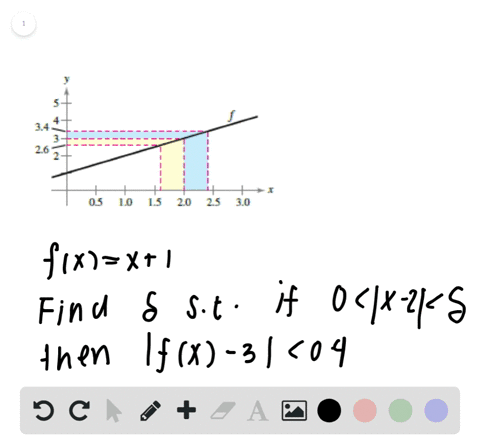Enroll in one of our FREE online STEM summer camps. Space is limited so join now!View Summer Courses### Finding a $\delta$ for a Given $\varepsilon$ The …

01:39

Need more help? Fill out this quick form to get professional live tutoring.

Get live tutoring
Problem 34

Sketching a Graph In Exercises 33 and $34,$ sketch a graph of a function $f$ that satisfies the given values. There are many correct answers.)

$$f(-2)=0$$
$$f(2)=0$$
$$\lim _{x \rightarrow-2} f(x)=0$$
$\lim _{x \rightarrow 2} f(x)$ does not exist.

## Discussion

You must be signed in to discuss.

## Video Transcript

in this problem, we was just sketched the graph of a function f that satisfies the following. We want that every negative too is equal to zero. We want the f of positive two is equal to zero that the limit as X approaches negative too is equal to zero and that the limit is X approaches positive to does not exist. So I would like to start off with the function values that we know we know, regardless of what the function, really. They look like that half of negative, too zero and so is awful, too. So we can kind of at least fill in those coordinates here to get us started. So as stated in the function, Um, I'm sorry in the question statement, there are many correct answers. Was problem infinitely many actually. So we just need to provide one of those. So the answer you get is a little different from this one. That's expected because there's a lot of different graphs you could draw that will satisfy these conditions. So So now we have one and two done, and now I have to take care of the limits. So we know that the limit is ex purchase Nick if 20 So that tells us right away that the limit exists and it's also equal two the value of the function there. So that's equal to half of negative, too. So that tells us our graph is going to look pretty smooth here so it can kind of do something like this. Essentially, we know there's no breaks in the graph there. So two more biscuit work. We were not told anything about any other X values. Besides, positive to civil kind of fill in the graph until you get here and then decide what to do. So now we know that the limit as X approaches positive to just don't exist. So that means this Lim is certainly not equal to f of positive, too. So we don't want to draw something smooth. But we want something where the limit one exist. So as X approaches positive too, we can make our craft go towards one of the infinities, or it can go in two different directions out and so on. So there's a few ways we can go about this. Hey, so to make the limit not exist here, we can make the left limit equal to two. And let's say the right Lim. It's approaching three. So since the left and right limits do not exist or sorry, do not agree, we would say the limit as X approaches to does not exist. So now we can see that all of our criteria are met, and this is one way to answer this question.